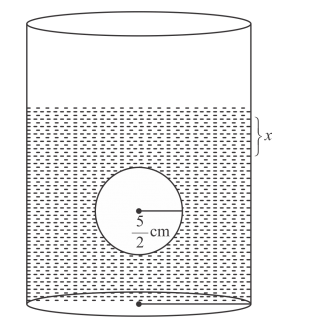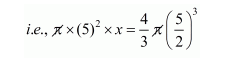# A sphere of diameter 5 cm is dropped into a cylindricalQuestion:

A sphere of diameter 5 cm is dropped into a cylindrical vessel partly filled with water. The diameter of the base of the vessel is 10 cm. If the sphere is completely submerged, by how much will the level of water rise?

Solution:

Radius of sphere $r=\frac{5}{2} \mathrm{~cm}$, radius of cylindrical vessel $r_{1}^{2}=\frac{10}{2} \mathrm{~cm}$.

When the sphere is completely submerged into the vessel. The level of water will be raised let x be height of level of raised water.Therefore,

The volume of raised water in cylindrical vessel = volume of sphere$25 x=\frac{4 \times 125}{3 \times 8}$

$x=\frac{4 \times 125}{3 \times 8 \times 25}$

$=\frac{5}{6} \mathrm{~cm}$

$x=\frac{5}{6} \mathrm{~cm}$

Hence, the level of water rise $=\frac{5}{6} \mathrm{~cm}$